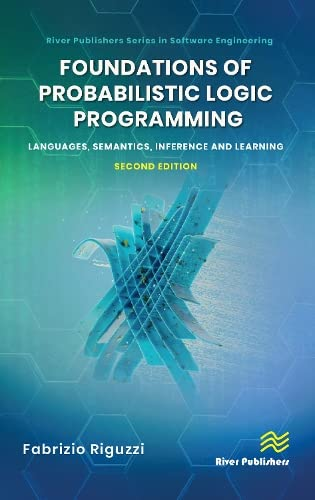# Foundations of Probabilistic Logic Programming: Languages, Semantics, Inference and Learning

• Length: 548 pages
• Edition: 2
• Publisher:
• Publication Date: 2023-07-07
• ISBN-10: 8770227195
• ISBN-13: 9788770227193
Description

Numerical methods are vital to the practice of chemical engineering, allowing for the solution of real-world problems. Written in a concise and practical format, this textbook introduces readers to the numerical methods required in the discipline of chemical engineering and enables them to validate their solutions using both Python and Simulink.

• Introduces numerical methods, followed by the solution of linear and nonlinear algebraic equations.
• Deals with the numerical integration of a definite function and solves initial and boundary value ordinary differential equations with different orders.
• Weaves in examples of various numerical methods and validates solutions to each with Python and Simulink graphical programming.
• Features appendices on how to use Python and Simulink.

Aimed at advanced undergraduate and graduate chemical engineering students, as well as practicing chemical engineers, this textbook offers a guide to the use of two of the most widely used programs in the discipline.

The textbook features numerous video lectures of applications and a solutions manual for qualifying instructors.